# Test: Hydroelectric Power Plant - 3

## 20 Questions MCQ Test Topicwise Question Bank for Mechanical Engineering | Test: Hydroelectric Power Plant - 3

Description
Attempt Test: Hydroelectric Power Plant - 3 | 20 questions in 60 minutes | Mock test for Mechanical Engineering preparation | Free important questions MCQ to study Topicwise Question Bank for Mechanical Engineering for Mechanical Engineering Exam | Download free PDF with solutions
QUESTION: 1

### An impulse turbine with a single nozzle has a specific speed of 6. What will be the approximate specific speed of the turbine if it is operated with three additional nozzle of the same size?

Solution:

Specific speed of multi-jet nozzle turbine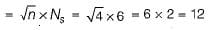QUESTION: 2

### Suggest the turbine that works under a head of 25 m, runs at 400 rpm and develops 16000 kW power. [Take 255/4 = 56]

Solution: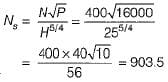So, the Kaplan turbine.

QUESTION: 3

### Select the wrong statement:

Solution:
QUESTION: 4

Consider the following statements pertaining to specific speed of machines:
1. Specific speed varies with shapes of the runner and other parts of the machine.
2. Machines with higher specific speeds are limited to low heads.
3. Specific speed is diamensionless and is independent of variation of type of fluid used.

Q. Which of the statements given above are correct?

Solution:
QUESTION: 5

If D is the diameter of Pelton wheel and d is the diameter of the jet, then number of buckets on the periphery of a Pelton wheel is equal to

Solution:
QUESTION: 6

Hydraulic turbines are directly coupled to the electric generators. The generators are always required to run at a constant speed irrespective of the variations in the load. The constant speed of the generator is given by

Solution: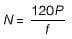where,
f is the frequency for power generated in cycles per second.
P is the number of poles for the generator.

QUESTION: 7

The specific speed of a turbine is given by the equation

Solution:
QUESTION: 8

The specific speed of a pump is given by the equation

Solution:
QUESTION: 9

Surge tank is used in pipe line

Solution:

Rapid velocity fluctuations occur in pipe line during
1. Sudden opening and closing of valves
2. Start and shut down of a turbine or closure of wicket gates during speed regulation of a turbine.
Due to rapid velocity fluctuations, large magnitude pressure transients are set-up and these excessive pressure may lead to bursting of pipe this phenomenon is known as water hammer. Some arrangement needs to be made to convert the rapid velocity fluctuations into slow velocity fluctuations and for this surge tank is provided just upstream of the power unit in a hydroelectric plant.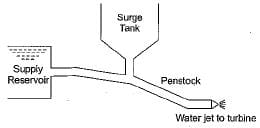QUESTION: 10

In axial flow turbine

Solution:

In axial flow turbine both inlet and exit of turbine is axial.
If water flows through the runner in the radial direction but leaves in the direction parallel to the axis of rotation of the runner. The turbine is called mixed flow turbine.

QUESTION: 11

Which one of the following turbine exhibits a nearly constant efficiency over a 60% to 140% of design speed

Solution:

A special type of propeller turbine is called Kaplan turbine in which the individual runner blades are Pivoted on the hub so that their inclination may be adjusted automatically rotating about Pivots with the help of a governor servo-mechanism.
In Kaplan turbine, because of the arrangement for automatic variation of inlet blade angle with variation in load, the turbine can be run at max efficiency at ail loads.

QUESTION: 12

The function of guide vanes in a reaction turbine is to

Solution:

Guide vanes are the vanes which are attached to the causing and guides the water to enter into the runner.

QUESTION: 13

The maximum number of Jets generally employed in impulse turbine without Jet interference is

Solution:
QUESTION: 14

A draft tube is used in reaction turbine

Solution:

It is a diverging tube which is attached at outlet of the runner to carry water from the exit of runner to tail race.
The diverging tube helps to increase the pressure on the account of conversion of kinetic head into pressure head.

QUESTION: 15

Which one of the following is an example of the pure (100%) reaction machine

Solution:
QUESTION: 16

Hydraulic efficiency of a turbine is defined as

Solution:

Hydraulic Efficiency: It is defined as the ratio of power given by the water to runner of a turbine (runner is a rotating part of a turbine and on the runner vanes are fixed) to the power supplied by the water at the inlet of the turbine.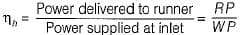QUESTION: 17

Operating characteristic curves of a turbine means

Solution:
QUESTION: 18

The following two relationship are necessary for homologous turbine units

Solution:
QUESTION: 19

Match List-I (Hydraulic turbine) with List-ll. (Application area) and select the correct answer using the codes given below the lists:
List - I
A. Pelton turbine
B. Francis turbine
C. Kaplan turbine
List - II
Codes:
A B C
(a) 2 3 1
(b) 2 1 3
(c) 3 1 2
(d) 3 2 1

Solution:

Pelton wheel: It is high head (1770 m) and low discharge turbine.
Francis turbine: It is medium head (60 - 250 m) and medium discharge turbine.
Kaplan Turbine: It is low head (less than 60 m) and high discharge turbine.

QUESTION: 20

As water flows through the runner of a reaction turbine, pressure acting on it would vary from

Solution:

At inlet of reaction turbine water is admitted with high pressure head (above atmospheric pressure) with some kinetic energy. The pressure head of water gradually decreases from inlet to exit. Finally water leaves the runner with low pressure and small kinetic energy to tail race. Quite often, the pressure head of the water at turbine exit fall below the ambient pressure. A draft tube attached to the exit of the turbine, working like a diffuser converts the remaining kinetic energy of water discharged by the runner into the pressure energy so that water is discharged to the tail race with a positive pressure head to avoid cavitation.Use Code STAYHOME200 and get INR 200 additional OFF Use Coupon Code